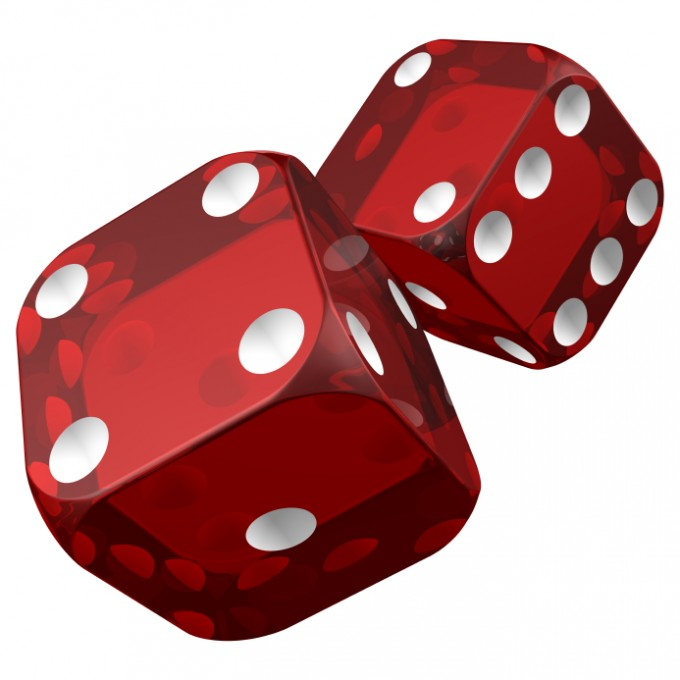## R Project - The Game of CrapsIn the game of Craps, a player rolls a pair of dice. If, on this first roll, the sum of the dice is 7 or 11, they win immediately. If the sum of the dice is 2, 3, or 12, they lose immediately. If instead, they roll some other value $x$ (called the "point"), they continue to roll the dice until either the point is rolled (where the player then wins), or a 7 is rolled (where the player then loses).

1. Write an R function craps() that simulates playing the game of craps and returns either "win" or "lose", as appropriate. You may find following the below outline useful to this end:

1. Simulate the sum of rolling 2 dice and save this value to a variable. This is your first roll.

2. The following three steps can be done using a while-loop combined with some conditional statements (either the simple if-statement, an if-else-statement, or even the ifelse() function). That said, it is probably most efficient to use a while loop combined only with simple if-statements.

• If you roll 7 or 11, then you win.
• If you roll 2, 3, or 12, then you lose.
• If you roll something else, keep rolling until you later roll value that will end the game (either 7 or the first roll's value). A while loop is useful for the "keep rolling" part. Finally, decide whether you won.

Answers vary, of course. Here's a fast way to write this function:
craps = function() {
first.roll = sum(sample(1:6,size=2,replace=TRUE))
if (any(c(7,11) == first.roll)) return("win")
if (any(c(2,3,12) == first.roll)) return("lose")
while (TRUE) {
roll = sum(sample(1:6,size=2,replace=TRUE))
if (roll == first.roll) return("win")
if (roll == 7) return("lose")
}
}


If you like using if-else conditionals with curly-braces, you can do that too (see below). However, the else's are not really necessary here as the action of returning a value stops the function from executing anything else, and the braces are not necessary as you are only ever doing a single statement as a result of a condition being true or false below.

craps = function() {
first.roll = sum(sample(1:6,size=2,replace=TRUE))
if (any(c(7,11) == first.roll)) return("win") else {
if (any(c(2,3,12) == first.roll)) return("lose") else {
while (TRUE) {
roll = sum(sample(1:6,size=2,replace=TRUE))
if (roll == first.roll) return("win") else {
if (roll == 7) return("lose") }
}
}
}
}

2. Write an R function simulated.probability.of.winning.craps() to estimate the probability of winning the game of craps. Use this to test your craps() function. The probability of winning craps should be approximately $0.493$.

simulated.probability.of.winning.craps = function() {
return(sum(replicate(10000,craps()) == "win")/10000)
}

3. As a variant on the above, write a function craps.dice.rolls() that returns the sequence of dice rolls along with the end result.

craps.dice.rolls = function() {
first.roll = sum(sample(1:6,size=2,replace=TRUE))
rolls = first.roll
if (any(c(7,11) == first.roll)) return(c(rolls,"win"))
if (any(c(2,3,12) == first.roll)) return(c(rolls,"lose"))
while (TRUE) {
roll = sum(sample(1:6,size=2,replace=TRUE))
rolls = c(rolls,roll)
if (roll == first.roll) return(c(rolls,"win"))
if (roll == 7) return(c(rolls,"lose"))
}
}